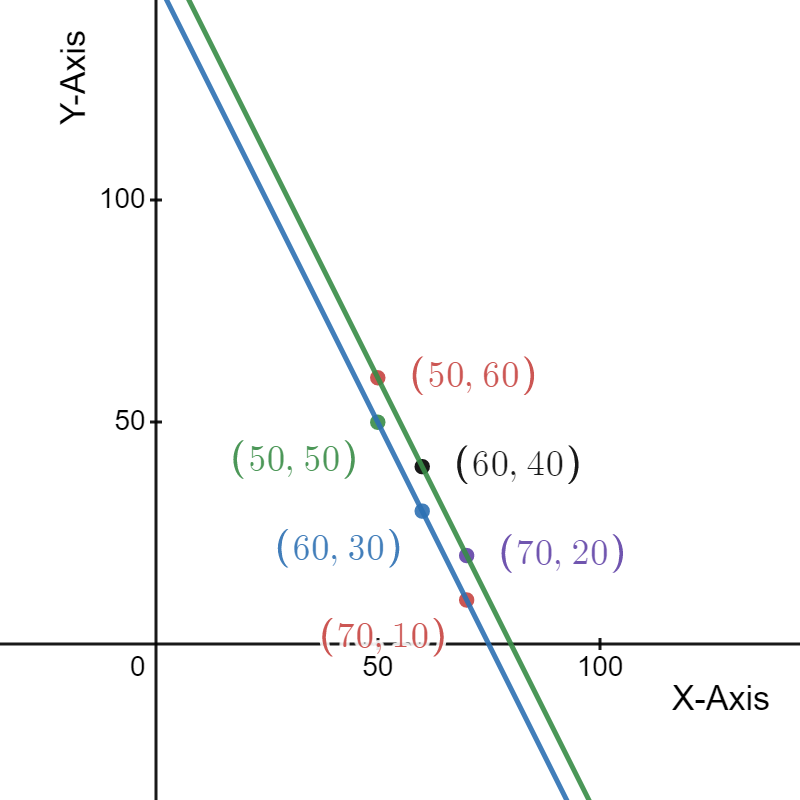The cost of 2 kg of apples and 1 kg grapes on a day was bound to be Rs. 160. After a month the cost of 4 kg apples and 2 kg grapes is Rs. 300. Represent this situation algebraically and geometrically. The two linear equations are perpendicular lines. Is it true or false?

# The cost of 2 kg of apples and 1 kg grapes on a day was bound to be Rs. 160. After a month the cost of 4 kg apples and 2 kg grapes is Rs. 300. Represent this situation algebraically and geometrically. The two linear equations are perpendicular lines. Is it true or false?

1. A
True
2. B
False

Fill Out the Form for Expert Academic Guidance!l

+91

Live ClassesBooksTest SeriesSelf Learning

Verify OTP Code (required)

### Solution:

Let us assume the cost of the one kg of apple be ‘x’
The cost of one kg of grapes is ‘y’.
Algebraic equation for 2 kg apple and 1 kg of grapesAlgebraic equation for 4kg apples and 2kg grapesSubtract the equation 2 and 1 we getFrom equation (1)X 60 50 7040 60 20
From equation (3)X 60 50 7030 50 10
Let us plot the system of linear equations using the obtained values of x and y-coordinates.Based on the graph, the two linear equations represent parallel lines.
Hence, the statement is false.

## Related content

 Area of Square Area of Isosceles Triangle Pythagoras Theorem Triangle Formula Perimeter of Triangle Formula Area Formulae Volume of Cone Formula Matrices and Determinants_mathematics Critical Points Solved Examples Type of relations_mathematics+91

Live ClassesBooksTest SeriesSelf Learning

Verify OTP Code (required)